# Math in Focus Grade 3 Chapter 10 Practice 7 Answer Key Real-World Problems: Money

Practice the problems of Math in Focus Grade 3 Workbook Answer Key Chapter 10 Practice 7 Real-World Problems: Money to score better marks in the exam.

## Math in Focus Grade 3 Chapter 10 Practice 7 Answer Key Real-World Problems: Money

Question 1.
Nick has $18.20. He buys a pencil case for$12.50. How much does he have left?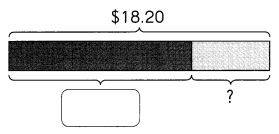$18.20 –$12.50 = $___________ He has$_________ left.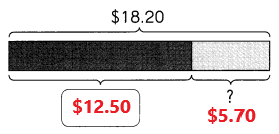$18.20 –$12.50 = $5.70 He has$5.70 left.
Explanation:
Nick has $18.20. He buys a pencil case for$12.50. Subtract $12.50 from$18.20 the difference is $5.70. He has$5.70 left.

Question 2.
Tim has $40. Tim’s sister has$17.25 more. How much does his sister have?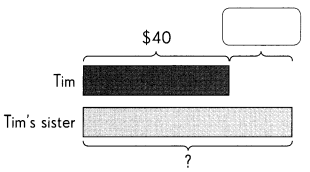$40 +$17.25 = $_________ Tim’s sister has$___________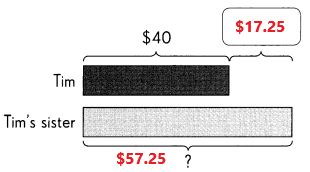$40 +$17.25 = $57.25 Tim’s sister has$57.25.
Explanation:
Tim has $40. Tim’s sister has$17.25 more. Add $40 with$17.25 the sum is $57.25. Tim’s sister has$57.25.

Question 3.
Pamela buys a dress for $35.70. She buys a pair of shoes that costs$3.20 more than the dress.
a. How much is the pair of shoes?

b. How much does she spend in all?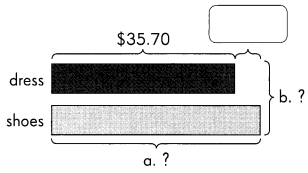a. $35.70 +$3.20 = $__________ The pair of shoes is$__________.
$35.70 +$3.20 = $38.90 The pair of shoes is$38.90.
Explanation:
Pamela buys a dress for $35.70. She buys a pair of shoes that costs$3.20 more than the dress. Add $35.70 with$3.20 the sum is $38.90. The pair of shoes is$38.90.

b. $35.70 +$ = $__________ She spends$__________ in all.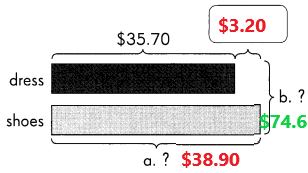$35.70 + 38.90 =$74.60
She spends $74.60 in all. Explanation: Pamela buys a dress for$35.70. She buys a pair of shoes that costs $38.90. Add$35.70 with $38.90 the sum is$74.60. She spends $74.60 in all. Question 4. Roberto has$7.85 to start with. Then he saves $2.40. He wants to buy a football that costs$16.70.
a. How much money does he have?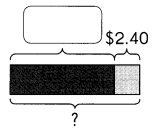$7.85 +$2.40 = $_________ He has$ _________.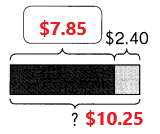$7.85 +$2.40 = $10.25 He has$10.25.
Roberto has $7.85 to start with. Then he saves$2.40. Add $7.85 with$2.40 the sum is $10.25. He has$10.25.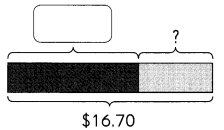$16.70 –$_________ = $_________ He must save$_________ more.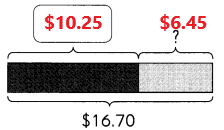$16.70 –$10.25 = $6.45 He must save$6.45 more.
He has $10.25. He wants to buy a football that costs$16.70. Subtract $10.25 from$16.70 the difference is $6.45. He must save$6.45 more.